Next: Projective Invariants Up: Important concepts from projective Previous: Manipulating Points and

# Projective Transformations

The idea of a projective plane can be applied to \$n\$-dimensional space in order to define projective n-space. Of particular interest is projective 3-space. Transformations within and between projective spaces are called projectivities and are the fundamental concern of projective geometry. Certain properties and measurements remain invariant under the action of a projectivity - invariant properties include collinearity, concurrency, tangency and incidence; invariant measurements, which are referred to as projective invariants.

There is no emphasis on projective spaces of any particular dimension in a purely mathematical study of projective geometry, but in computer vision some cases are of more interest than others. We consider two projectivities in the rest of the section - perspective projection to the image plane, and plane-to-plane projection - and in doing so cover some general points.

(a) Perspective projection to the image plane.

Perspective projection is a projectivity from projective 3-space to the projective plane. It has the formwhereare the homogeneous coordinates of a point on the image plane,is a 3-by-4 matrix, andare the homogeneous coordinates of a point in the world.

Perspective projection is a particular type of projectivity called a perspectivity, in which all rays of projection pass through a single point - this puts constraints on the form of the matrix P as described in [Mundy 1992].

(b) Plane-to-plane projection.

A projectivity from a projective plane to a projective plane is called a plane-to-plane projectivity, although it is often referred to by simply using the more general term of projectivity. It acts on, and generates, a homogeneous 3-vector and is therefore a 3-by-3 matrix.

To see how such a projectivity arises, consider two images taken from different viewpoints of a plane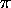in a scene, Figure 1. The mapping of points onto the corresponding points in image 1 is described by a projectivity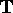. Similarly, the mapping of points onto the corresponding points in image 2 is described by a projectivity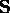. An important property of projectivities is that they form a group. It follows that there is a projectivitywhich describes the mapping of the image ofin image 1 to the image ofin image 2, where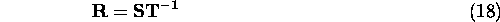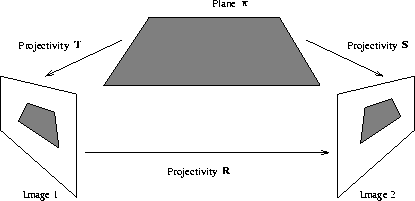Figure: Two images are taken of the planein the scene. There is a projectivity between each image and the plane, and between the image ofin image 1 and the image ofin image 2.

Chapter 2 contains an example of a projectivity computed between one image and another, and Chapter 8 an example of a projectivity computed between an image and a plane in the scene.

Computation of a projectivity such asrequires four points ontogether with their mapped points in the image. The following method of computation is given in [Rothwell 1992]. Let the projectivity map point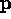to point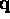. These pointsandare normalised by scaling them so that their third components are equal to one. The scale ofis arbitrary and is set by making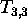equal to one. Then the mapping oftois described bywhere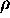is a scale factor.

The matrix equation (19) yields three linear equations, and eliminating the unknown scale factorleaves two linear equations in the eight unknowns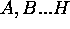,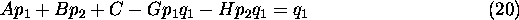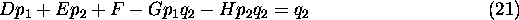Thus four pairs of points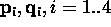, give eight linear equations in the eight unknowns and it is possible to solve for. The condition for linear independence of the equations is that no three of the four points are collinear. Given more than four pairs of points, the system can be solved using a least-squares method such as the pseudo-inverse.

Since there is a duality between points and lines on the projective plane, it is natural to ask ifalso describes the mapping of lines. In fact, it is simple to show [Mundy 1992] that ifis a point projectivity describing the mapping of points from projective plane 1 to projective plane 2, then the line projectivity describing the mapping of lines from projective plane 1 to projective plane 2 is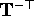(the inverse transpose of). Also, the inverse point projectivity for the mapping of points from projective plane 2 to projective plane 1 is, and the inverse line projectivity is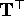(existence of the inverse is guaranteed because projectivities form a group).

(c) Extra discussion on the plane-to-plane projectivity.

There are some problems with the method in (b) for computing a plane-to-plane projectivity. The normalisation stage, where the homogeneous vectors are scaled so that their third component is one, andis set to one, is carried out to make the equations linear. This is inappropriate if the elements which are being normalised are actually of value zero. (It is possible to detect when the third component of a homogeneous vector is zero and remove it from the processing, but there is no way to trap the case ofbeing zero because this is one of the unknowns in the system). Although these failure conditions did not cause difficulty in practice in the work in this thesis, an alternative method for computing the projectivity would be preferable. A possible approach is to find the projectivitywhich minimises the expressionwhere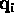is the desired position to which a point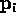is to be mapped. (There will always be noise in a real system, so there is unlikely to be awhich maps allexactly to the corresponding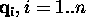. Equation (22) identifies thewhich maps the``as closely as possible'' to the corresponding.) The scale ofis arbitrary, so Equation (22) is used in conjunction with a normalisation condition such as,This approach overcomes the problems associated with the method in (b) at the expense of being a non-linear system.Next: Projective Invariants Up: Important concepts from projective Previous: Manipulating Points and

Bob Fisher
Fri Nov 7 12:08:26 GMT 1997